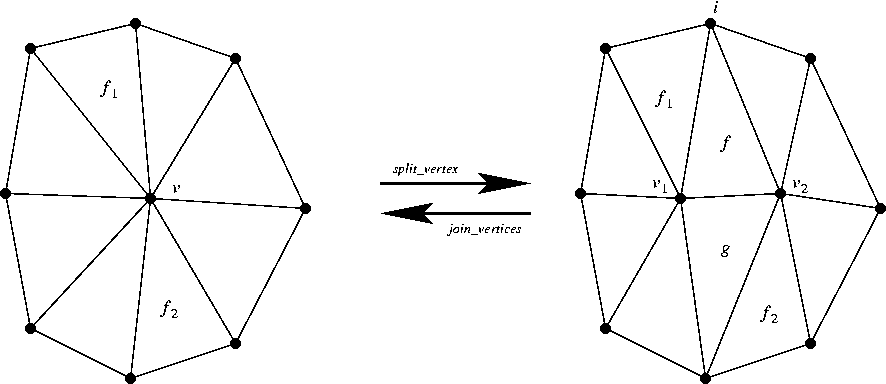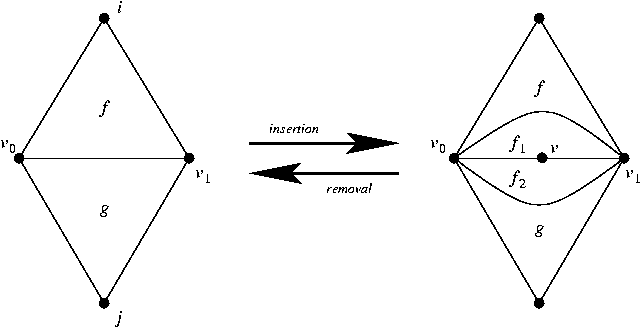## CGAL::Triangulation_data_structure_2<Vb,Fb>

### Definition

The class Triangulation_data_structure_2<Vb,Fb> is a model for the TriangulationDataStructure_2 concept. It can be used to represent an orientable 2D triangulation embedded in a space of any dimension.

#include <CGAL/Triangulation_data_structure_2.h>

### Is Model for the Concept

TriangulationDataStructure_2

### Modifiers

In addition to the modifiers required by the TriangulationDataStructure_2 concept, the Triangulation_data_structure_2<Vb,Fb> class supports also the modifiers below. Note also that the modifiers below guarantee the combinatorial validity of the resulting data structure.

Vertex_handle tds.join_vertices ( Face_handle f, int i)
Joins the vertices that are endpoints of the edge (f,i). It returns a vertex handle to common vertex (see Fig.).
Precondition: f must be different from Face_handle() and i must be 0, 1 or 2.
Vertex_handle tds.join_vertices ( Edge e)
Joins the vertices that are endpoints of the edge e. It returns a vertex handle to common vertex.
Vertex_handle tds.join_vertices ( Edge_iterator eit)
Joins the vertices that are endpoints of the edge *eit. It returns a vertex handle to common vertex.
Vertex_handle tds.join_vertices ( Edges_circulator ec)
Joins the vertices that are endpoints of the edge *ec. It returns a vertex handle to common vertex.
boost::tuples::tuple<Vertex_handle, Vertex_handle, Face_handle, Face_handle>
 tds.split_vertex ( Vertex_handle v, Face_handle f1, Face_handle f2)
Splits the vertex v into two vertices v1 and v2. The common faces f and g of v1 and v2 are created after (in the counter-clockwise sense) the faces f1 and f2. The 4-tuple (v1,v2,f,g) is returned (see Fig.).
Precondition: dimension() must be equal to 2, f1 and f2 must be different from Face_handle() and v must be a vertex of both f1 and f2.

Vertex_handle tds.insert_degree_2 ( Face_handle f, int i)
Inserts a degree two vertex and two faces adjacent to it that have two common edges. The edge defined by the face handle f and the integer i is duplicated. It returns a handle to the vertex created (see Fig.).

void tds.remove_degree_2 ( Vertex_handle v)
Removes a degree 2 vertex and the two faces adjacent to it. The two edges of the star of v that are not incident to it are collapsed (see Fig.).
Precondition: The degree of v must be equal to 2.Figure:  The join and split operations.Figure:  Insertion and removal of degree 2 vertices.